# Create Nets of 3 Dimensional Figures to Find the Surface Area: A 6th Grade Geometry Lesson

Page content

A net is a two-dimensional drawing of a three-dimensional figure. Students will represent a three-dimensional figure a net composed of rectangles and triangles. Using the measurements of the two-dimensional figures of the net, students will determine the area of each rectangle and/or triangle. Then, find the sum of the areas of the net to determine the surface area of the three-dimensional figure.

Lesson Objective: The lesson is aligned to the Common Core State Standards for Mathematics – 6.G.4 Geometry – Represent three-dimensional figures using nets made up of rectangles and/or triangles, and use the nets to find the surface area of these figures.

Materials Required: Graph paper, calculator

## Lesson Procedure:

**Finding the Surface Areas of Three-Dimensional Figures
**

To find the surface area of a three-dimensional figure, use the following steps:

1. Draw a net of the three-dimensional-figure.
2. Find the area of each face of the net.
3. To find the surface area of the cube, find the sum of the area of all the faces of the net.

Look at the cube shown on the right. The measurements of the sides of the cube are 2 inches.

1. Draw a net of the cube. There are six faces in the net. Each face is a square. Label the dimensions of each face.
2. Find the area of each face of the net. What is the area of each face?
3. To find the surface area of the cube, find the sum of the area of all of the faces of the net. What is the surface area of the cube?

1.The net of the cube. Each side has a measurement of 2 inches.

2. There are 6 faces that are in the shape of a square. Each face has an area of 4 square inches.

3. The surface area of the cube is 6 x 4 = 24 square inches.

## Individual or Group Work:

Use the three steps shown above to find the surface area of the three-dimensional figures.

1. What is the surface area of the rectangular prism?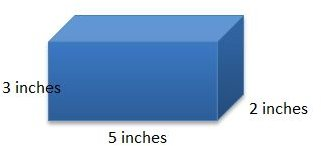2. A square pyramid has the measurements shown below. What is the surface are aof the pyramid? Note: s refers to the slant height–the height of the traingles. b = 6 inches. h = 4 inches. s = 5 inches. What is the surface area of the square pyramid?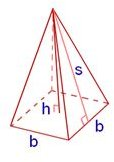3. What is the surface area of the cube?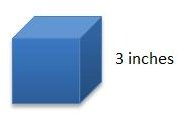4. A square pyramid has the measurements shown below. What is the surface area of the pyramid? Note: s refers to the slant height – the height of the trianbles. b = 5 inches. h = 7.6 inches. s = 8 inches.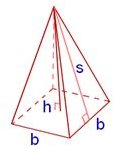5. Find the surface area of the rectangular prism.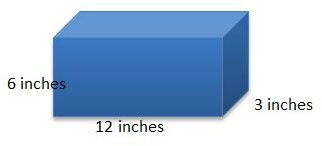1. The net of the rectangular prism is 6 rectangles, 4 rectangles with dimensions 3 x 5 and 2 rectangles with dimensions 2 x 3. The area of each of the 4 rectangles is l w, 3 x 5 = 15 square inches. The area of each of the 2 rectangles is 2 x 3 = 6 square inches. The surface area of the rectangular prism is 4 (15) + 2 (6) = 72 square inches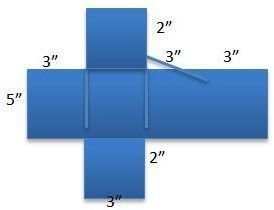2. The net of the square pyramid is 1 square and 4 triangles. The area of the square is b squared, 36 square inches. The area of each triangle is 1/2 b x s, 15 square inches. The surface area of the square pyramid is 36 + 4 x 15 = 36 + 60 = 96 square inches.

3. The net of the cube is 6 squares with each side measuring 3 inches. The area of one square is 9 square inches. The surface area of the cube is 6 (9) = 54 square inches.

4. The net of the square pyramid is 1 square and 4 triangles. The area of the square is b squared, 25 square inches. The area of each triangle is 1/2 b x s, 20 square inches. The surface area of the square pyramid is 25 + 4 x 20 = 25 + 80 = 105 square inches.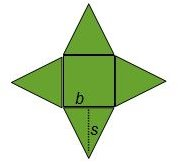5. The net of the rectangular prism is 6 rectangles, 4 rectangles with dimensions 6 x 12 and 2 rectangles with dimensions 3 x 6. The area of each of the 4 rectangles is 6 x 12 = 72 square inches. The area of each of the 2 rectangles is 3 x 6 = 18 square inches. The surface aea of the rectangular prism is 4 ( 72) + 2 (18) = 324 square inches.